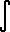Home    |    Teacher    |    Parents    |    Glossary    |    About UsEmail this page to a friendResources· Cool Tools · Formulas & Tables · References · Test Preparation · Study Tips · Wonders of MathSearchProof: Integral bx (Math | Calculus | Integrals | Table Of | bx)

 Discussion ofbx dx = bx / ln(b) + C.
1. Proof
Strategy: Use theeu du = eu + C

since eln b = b,bx dx =[ (eln b) x ] dxe (ln b) x dx

set u = (ln b) x
then du = (ln b) dx
substitute...eu (du / ln b)
= (1 / ln b)eu du

solve the integral...

= (1 / ln b) ( eu + C )
= (1 / ln b) eu + C2   (create new constant)

substitute back u = (ln b) x,

= ( 1 / ln b) e(ln b) x + C2
= ( 1 / ln b) ( e(ln b) )x + C2
= ( 1 / ln b) bx + C2
= bx / ln b + C2
Q.E.D.

See also the proof ofeu du = euPROOF

2. You need not memorize this theorem.  Derive it each time you use it.
Consider this example: if you have the integral:2x dx.
There is no need to memorize the formula.  We will get this integral into the easier form,eu du.

Recall that eln(2) = 22x dx =( eln (2) ) x dx
=eln (2) x dx

set u = ln(2) x
then du = ln(2) dx
substitute:eu (du / ln 2 )
= (1 / ln 2)eu du
= (1 / ln 2) eu + C
substitute back...
= (1 / ln 2) eln(2) x + C
= (1 / ln 2) ( eln(2) )x + C
= (1 / ln 2) 2x + C  ANSWER

This method is actually quite fast; it just looks long because I drew it out for demonstration purposes.